# Double Series

## double series

[¦dəb·əl ′sir‚ēz]
(mathematics)
A two-dimensional array of numbers whose sum is the limit of Sm, n, the sum of the terms in the rectangular array formed by the first n terms in each of the first m rows, as m and n increase.

## Double Series

an expression of the form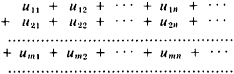composed of the elements of an infinite matrix ǀǀumn ǀǀ (m, n = 1,2,...). These elements may be numbers (then the double series is called a double series of numbers) or functions of one or several variables (double series of functions). An abbreviated notation is used for the double series: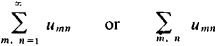umnis called the general term of the double series. The finite sums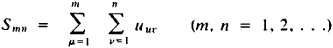are called partial sums of the double series. If the limit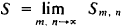exists when m and n tend to infinity independently from each other, then this limit is said to be the sum of the double series and the double series is said to be convergent. The theory of the convergence of double series is considerably more complex than the corresponding theory for simple series; for example, in contrast to the simple series, the convergence of a double series does not imply that its partial sums are bounded.

The expression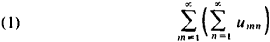is called a repeated series. Here we are required to sum first the series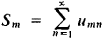composed of the sums Sm , If the repeated series (1) is convergent and has theis convergent and has the sum S, then it is called the row sum of the double series. The column sum S’ of the double series is defined in an analogous manner. The convergence of the double series does not imply the convergence of the series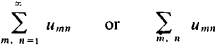and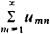so that the row sums and column sums may not even exist. Conversely, if the double series diverges, it may turn out that the sums over the row sums and column sums exist and that S ≠ S. However, if the double series converges and has the sum S and if the row sum and column sums exist, then each of these sums is equal to 5. This fact is always used in the actual calculation of the sum of a double series.

The most important classes of the double series are the double power series, the double Fourier series, and the quadratic forms with an infinite number of variables. For the double Fourier series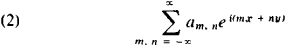one of the standard concepts concerning the sum of such series is the following: we form the circular (or spherical) partial sums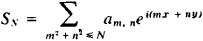where the summation is over all pairs of integers (m,n) for which m2 + n2N, and then consider limit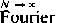. This limit is called the spherical sum of the double Fourier series (2). Many important functions are represented with the aid of double series, for example, Weierstrass’ elliptic function.

A multiple series (more precisely, an s-multiple series) is an expression of the form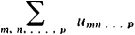constructed from the members of the table ǀǀ umn . . p ǀǀ. Each member of this table has s indexes m, n, . . . , p, and these indexes run independently through all the natural numbers. The theory of multiple series is completely analogous to the theory of double series.

### REFERENCES

Fikhtengol’ts, G. M. Kurs differentsial’nogo i integral’ nogo ischislenia, 6th ed., vol. 2. Moscow, 1966.

S. B. STECHKIN

Mentioned in ?
References in periodicals archive ?
Multiple World Champions will be competing against each other, including Great Britain's double series winner, Tai Woffinden.
The Indian women's team are fresh from their victory against South Africa, where they scripted history by clinching a double series win in a single tour for the first time ever.
Then, the sum of a double series [[summation].sub.i,j] [x.sub.ij] relating to this rule is defined by [??] - [[summation].sub.i,j][x.sub.ij] = [??] - [lim.sub.m,n[right arrow][infinity]] [[summation].sup.m,n.sub.i,j=0] [x.sub.ij].
Compared with [26, 27, 30], the random response of this paper for the stochastic van der Pol system is directly expressed as the double series form of deterministic response using the known orthogonal polynomial functions by means of the sequential orthogonal decomposition.
It details the elementary theory of infinite series; the basic properties of Taylor and Fourier series, series of functions, and the applications of uniform convergence; double series, changes in the order of summation, and summability; power series and real analytic functions; and additional topics in Fourier series, such as summability, Parseval's equality, and the convolution theorem.
Victory in the opening round of the six-stage series sets the double series winners up well for a hat-trick this season, where the top four teams qualify for rugby's Olympic debut in 2016.
Recently, Altay and Basar (2005), have defined the spaces BS, BS (t), [CS.sub.p], [CS.sub.bp] [CS.sub.r] and BV of double sequences consisting of all double series whose sequence of partial sums are in the spaces [M.sub.u], Vm(t), [C.sub.p], [C.sub.bp], [C.sub.r] and [[Laplace].sub.u] respectively, and also examined some properties of those sequence spaces and determined the [alpha]--duals of the spaces BS, [CS.sub.bp] and BV and the [omicron] [beta] (I)--duals of the spaces [CS.sub.bp], [CS.sub.r] of double series.
More recently, Altay and Basar  have defined the spaces BS, BS (t), [CS.sub.p], [CS.sub.bp], [CS.sub.r] and BV of double sequences consisting of all double series whose sequence of partial sums are in the spaces [M.sub.u], [M.sub.u](t), [C.sub.p], [C.sub.bp], [C.sub.r] and [L.sub.u], respectively, and also have examined some properties of those sequence spaces and determined the [alpha]-duals of the spaces BS, BV, [CS.sub.bp] and the [beta]([??])-duals of the spaces [CS.sub.bp] and [CS.sub.r] of double series.
Hence, letting p [right arrow] [infinity] in (12)-(14) and considering the absolute convergence of the obtained double series, we get the following theorem.
This yields a double series [summation over (m,n)] as for [I.sub.1] in Lemma 4.1 involving the integral [[integral].sup.T.sub.- T](m[[[(2n).sup.2] - [D.sup.2])].sup.it] dt.
Since Pringsheim introduced the notion of convergence of a numerical double series in terms of the convergence of the double sequence of its rectangular partial sums in , several authors have contributed to this topic during the last century.

Site: Follow: Share:
Open / Close